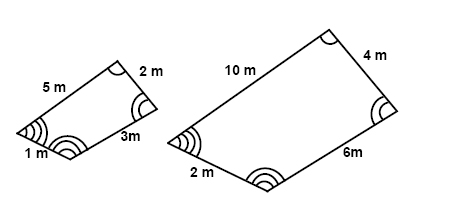# Polygons

Any two polygons are similar if their corresponding angles are congruent and the measures of their corresponding sides are proportional:In the figure above the ratio or the scale factor of the quadrilateral to the left versus the quadrilateral to the right is ½. We could also turn it around and say that the scale factor for the quadrilateral to the right to the quadrilateral to the left is 2.

## Video lesson

Find the ratio between the two similar figures